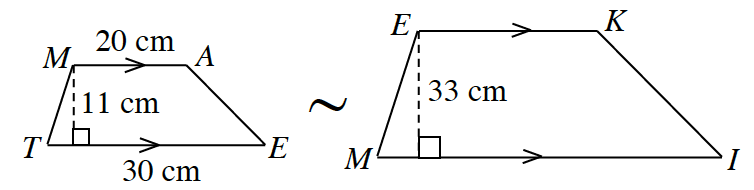### Home > CCG > Chapter 4 > Lesson 4.1.5 > Problem4-47

4-47.The trapezoids at right are similar.

Refer to the Math Notes box in Lesson 3.1.2.

Use proportional equations to find the missing dimensions.
Refer to the Math Notes box in Lesson 3.1.3.

The formula to find the area of a trapezoid is $\frac{1}{2}h(b_1+b_2)$

1. What is the ratio of the heights?

2. Compare the areas. What is the ratio of the areas?